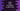# Swift program to check if a number is odd or even## How to find if a number is even or odd in Swift:

A number is called an even number if it is divisible by 2, else it is odd. If you want to check if a number is odd or even, you can simply use the modulo `%` operator in Swift. We can also find out the remainder by using the `isMultiple` and `remainder` methods. In this post, I will show you how to iterate through the elements of an integer array and how to check odd/even for each number in that array.

### Method 1: By using the modulo operator:

The modulo operator returns the remainder by dividing one number by a different number. The following example uses the modulo operator, `%` to check if a number is even or not:

``````var arr = [1,2,3,4,5,6]

for n in arr{
if(n % 2 == 0){
print("\(n) is even")
}else{
print("\(n) is odd")
}
}``````

In this example, the program is checking all numbers of `arr`. It uses a `for` loop and uses the modulo operator `%` to check if the numbers are even or odd. It prints one message if the number is even or odd.

### Output :

If you run the above program, it will print the following output:

``````1 is odd
2 is even
3 is odd
4 is even
5 is odd
6 is even``````

### Method 2: By using the `isMultiple` method:

The `isMultiple` is an instance method that returns one boolean value if a number is multiple of another number. The `isMultiple` method is defined as:

``func isMultiple(of other: Self) -> Bool``

It returns `true` if the number is multiple of another number, else it returns `false`.

``````var arr = [1,2,3,4,5,6]

for n in arr{
if(n.isMultiple(of: 2)){
print("\(n) is even")
}else{
print("\(n) is odd")
}
}``````

It prints the same output.

### Method 3: How to use the `remainder` method with doubles:

Swift double provides the `remainder` instance method to find out the remainder of a division. The method is declared as:

``func remainder(dividingBy other: Self) -> Self``

It divides a number by a different number `other` and returns the remainder. The following example shows how it works:

``````var arr:[Double] = [1,2,3,4,5,6]

for n in arr{
if(n.remainder(dividingBy: 2) == 0){
print("\(n) is even")
}else{
print("\(n) is odd")
}
}``````

``````1.0 is odd